x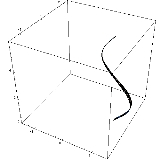Solid of revolutionOverview

In mathematics
Mathematics
Mathematics is the study of quantity, space, structure, and change. Mathematicians seek out patterns and formulate new conjectures. Mathematicians resolve the truth or falsity of conjectures by mathematical proofs, which are arguments sufficient to convince other mathematicians of their validity...

, engineering
Engineering
Engineering is the discipline, art, skill and profession of acquiring and applying scientific, mathematical, economic, social, and practical knowledge, in order to design and build structures, machines, devices, systems, materials and processes that safely realize improvements to the lives of...

, and manufacturing
Manufacturing
Manufacturing is the use of machines, tools and labor to produce goods for use or sale. The term may refer to a range of human activity, from handicraft to high tech, but is most commonly applied to industrial production, in which raw materials are transformed into finished goods on a large scale...

, a solid of revolution is a solid figure
Shape
The shape of an object located in some space is a geometrical description of the part of that space occupied by the object, as determined by its external boundary – abstracting from location and orientation in space, size, and other properties such as colour, content, and material...

obtained by rotating a plane curve
Plane curve
In mathematics, a plane curve is a curve in a Euclidean plane . The most frequently studied cases are smooth plane curves , and algebraic plane curves....

around some straight line (the axis) that lies on the same plane.

Assuming that the curve does not cross the axis, the solid's volume
Volume
Volume is the quantity of three-dimensional space enclosed by some closed boundary, for example, the space that a substance or shape occupies or contains....

is equal to the length
Length
In geometric measurements, length most commonly refers to the longest dimension of an object.In certain contexts, the term "length" is reserved for a certain dimension of an object along which the length is measured. For example it is possible to cut a length of a wire which is shorter than wire...

of the circle
Circle
A circle is a simple shape of Euclidean geometry consisting of those points in a plane that are a given distance from a given point, the centre. The distance between any of the points and the centre is called the radius....

described by the figure's centroid
Centroid
In geometry, the centroid, geometric center, or barycenter of a plane figure or two-dimensional shape X is the intersection of all straight lines that divide X into two parts of equal moment about the line. Informally, it is the "average" of all points of X...

multiplied by the figure's area
Area
Area is a quantity that expresses the extent of a two-dimensional surface or shape in the plane. Area can be understood as the amount of material with a given thickness that would be necessary to fashion a model of the shape, or the amount of paint necessary to cover the surface with a single coat...

(Pappus's second centroid Theorem
Pappus's centroid theorem
In mathematics, Pappus' centroid theorem is either of two related theorems dealing with the surface areas and volumes of surfaces and solids of revolution....

).

A representative disk is a three-dimension
Dimension
In physics and mathematics, the dimension of a space or object is informally defined as the minimum number of coordinates needed to specify any point within it. Thus a line has a dimension of one because only one coordinate is needed to specify a point on it...

al volume element
Volume element
In mathematics, a volume element provides a means for integrating a function with respect to volume in various coordinate systems such as spherical coordinates and cylindrical coordinates...

of a solid of revolution.Unanswered QuestionsHow to find the centroid of a cone?Encyclopedia
In mathematics
Mathematics
Mathematics is the study of quantity, space, structure, and change. Mathematicians seek out patterns and formulate new conjectures. Mathematicians resolve the truth or falsity of conjectures by mathematical proofs, which are arguments sufficient to convince other mathematicians of their validity...

, engineering
Engineering
Engineering is the discipline, art, skill and profession of acquiring and applying scientific, mathematical, economic, social, and practical knowledge, in order to design and build structures, machines, devices, systems, materials and processes that safely realize improvements to the lives of...

, and manufacturing
Manufacturing
Manufacturing is the use of machines, tools and labor to produce goods for use or sale. The term may refer to a range of human activity, from handicraft to high tech, but is most commonly applied to industrial production, in which raw materials are transformed into finished goods on a large scale...

, a solid of revolution is a solid figure
Shape
The shape of an object located in some space is a geometrical description of the part of that space occupied by the object, as determined by its external boundary – abstracting from location and orientation in space, size, and other properties such as colour, content, and material...

obtained by rotating a plane curve
Plane curve
In mathematics, a plane curve is a curve in a Euclidean plane . The most frequently studied cases are smooth plane curves , and algebraic plane curves....

around some straight line (the axis) that lies on the same plane.

Assuming that the curve does not cross the axis, the solid's volume
Volume
Volume is the quantity of three-dimensional space enclosed by some closed boundary, for example, the space that a substance or shape occupies or contains....

is equal to the length
Length
In geometric measurements, length most commonly refers to the longest dimension of an object.In certain contexts, the term "length" is reserved for a certain dimension of an object along which the length is measured. For example it is possible to cut a length of a wire which is shorter than wire...

of the circle
Circle
A circle is a simple shape of Euclidean geometry consisting of those points in a plane that are a given distance from a given point, the centre. The distance between any of the points and the centre is called the radius....

described by the figure's centroid
Centroid
In geometry, the centroid, geometric center, or barycenter of a plane figure or two-dimensional shape X is the intersection of all straight lines that divide X into two parts of equal moment about the line. Informally, it is the "average" of all points of X...

multiplied by the figure's area
Area
Area is a quantity that expresses the extent of a two-dimensional surface or shape in the plane. Area can be understood as the amount of material with a given thickness that would be necessary to fashion a model of the shape, or the amount of paint necessary to cover the surface with a single coat...

(Pappus's second centroid Theorem
Pappus's centroid theorem
In mathematics, Pappus' centroid theorem is either of two related theorems dealing with the surface areas and volumes of surfaces and solids of revolution....

).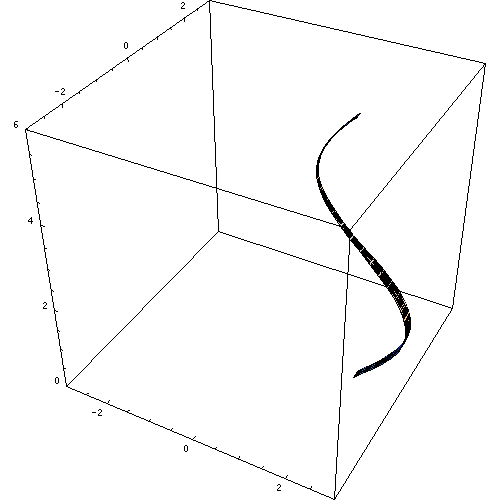A representative disk is a three-dimension
Dimension
In physics and mathematics, the dimension of a space or object is informally defined as the minimum number of coordinates needed to specify any point within it. Thus a line has a dimension of one because only one coordinate is needed to specify a point on it...

al volume element
Volume element
In mathematics, a volume element provides a means for integrating a function with respect to volume in various coordinate systems such as spherical coordinates and cylindrical coordinates...

of a solid of revolution. The element is created by rotating
Rotation
A rotation is a circular movement of an object around a center of rotation. A three-dimensional object rotates always around an imaginary line called a rotation axis. If the axis is within the body, and passes through its center of mass the body is said to rotate upon itself, or spin. A rotation...

a line segment
Line segment
In geometry, a line segment is a part of a line that is bounded by two end points, and contains every point on the line between its end points. Examples of line segments include the sides of a triangle or square. More generally, when the end points are both vertices of a polygon, the line segment...

(of length
Length
In geometric measurements, length most commonly refers to the longest dimension of an object.In certain contexts, the term "length" is reserved for a certain dimension of an object along which the length is measured. For example it is possible to cut a length of a wire which is shorter than wire...

w) around some axis (located r units away), so that a cylindrical
Cylinder (geometry)
A cylinder is one of the most basic curvilinear geometric shapes, the surface formed by the points at a fixed distance from a given line segment, the axis of the cylinder. The solid enclosed by this surface and by two planes perpendicular to the axis is also called a cylinder...

volume
Volume
Volume is the quantity of three-dimensional space enclosed by some closed boundary, for example, the space that a substance or shape occupies or contains....

of πr2w units is enclosed.

## Finding the volume

Two common methods for finding the volume of a solid of revolution are the disc method and the shell method of integration. To apply these methods, it is easiest to draw the graph in question; identify the area that is to be revolved about the axis of revolution; determine the volume of either a disc-shaped slice of the solid, with thickness δx, or a cylindrical shell of width δx; and then find the limiting sum of these volumes as δx approaches 0, a value which may be found by evaluating a suitable integral.

### Disc method

The disc method is used when the slice that was drawn is perpendicular to the axis of revolution; i.e. when integrating parallel to the axis of revolution.

The volume of the solid formed by rotating the area between the curves of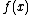and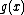and the linesand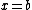about the x-axis is given by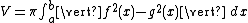If g(x) = 0 (e.g. revolving an area between curve and x-axis), this reduces to: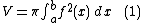The method can be visualized by considering a thin vertical rectangle at x between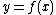on top and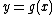on the bottom, and revolving it about the x-axis; it forms a ring (or disc in the case that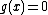), with outer radius f(x) and inner radius g(x). The area of a ring is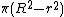, where R is the outer radius (in this case f(x)), and r is the inner radius (in this case g(x)). Summing up all of the areas along the interval gives the total volume. Alternatively, where each disc has a radius of f(x), the discs approach perfect cylinders as their height dx approaches zero. The volume of each infinitesimal disc is therefore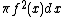. An infinite sum of the discs between a and b manifests itself as integral (1).

### Cylinder method

The cylinder method is used when the slice that was drawn is parallel to the axis of revolution; i.e. when integrating perpendicular to the axis of revolution.

The volume of the solid formed by rotating the area between the curves ofandand the linesandabout the y-axis is given by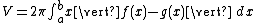If g(x) = 0 (e.g. revolving an area between curve and x-axis), this reduces to: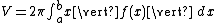The method can be visualized by considering a thin vertical rectangle at x with height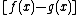, and revolving it about the y-axis; it forms a cylindrical shell. The lateral surface area of a cylinder is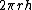, where r is the radius (in this case x), and h is the height (in this case). Summing up all of the surface areas along the interval gives the total volume.

## Parametric form

When a curve is defined by its parametric form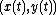in some interval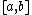, the volumes of the solids generated by revolving the curve around the x-axis, resp. the y-axis are given by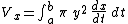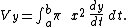Under the same circumstances the areas of the surfaces of the solids generated by revolving the curve around the x-axis, resp. the y-axis are given by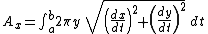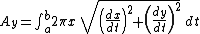## See also

• Gabriel's Horn
Gabriel's Horn
Gabriel's Horn is a geometric figure which has infinite surface area but encloses a finite volume. The name refers to the tradition identifying the Archangel Gabriel as the angel who blows the horn to announce Judgment Day, associating the divine, or infinite, with the finite...

• Guldinus theorem
• Pseudosphere
Pseudosphere
In geometry, the term pseudosphere is used to describe various surfaces with constant negative gaussian curvature. Depending on context, it can refer to either a theoretical surface of constant negative curvature, to a tractricoid, or to a hyperboloid....

• Surface of revolution
Surface of revolution
A surface of revolution is a surface in Euclidean space created by rotating a curve around a straight line in its plane ....

## External links

The source of this article is wikipedia, the free encyclopedia.  The text of this article is licensed under the GFDL.